The goal of riskviewer is to provide a modern implementation of the “Risk Characterisation Theatre” originally proposed by Erik Rifkin and Edward Bouwer in their 2007 book “The illusion of certainty: Health Benefits and Risks”.

## Why is this useful?

• uncertainty, embedded
• instinctive understanding of risk assessment figures based on real-world-scenarios

More context in the package vignette.

## Preliminary notes

The package has been created as a quick experimentation of how it would be possible to implement the risk characterisation theatre. At this stage, the codebase is still messy and poorly documented. Customisation of parameters is largely untested, so anything differing significantly from the examples documented in the README and vignette will likley not work. The package will however be improved in the coming weeks and months.

## Installation

You can install riskviewer from GitHub with:

# install.packages("remotes")
remotes::install_github("EDJNet/riskviewer)

## Examples

At this stage, this package provides two formats for representing risk.

### Airplane

library("riskviewer")
library("patchwork")
plane_1 <- rv_create_airplane(risk_ratio = 0.1,
rows = 33,
title = "Scenario A",
font_family = "Roboto Condensed",
font_family_seats = "Roboto Mono")

plane_2 <- rv_create_airplane(risk_ratio = 0.3,
rows = 33,
title = "Scenario B",
font_family = "Roboto Condensed",
font_family_seats = "Roboto Mono")

plane_1 + plane_2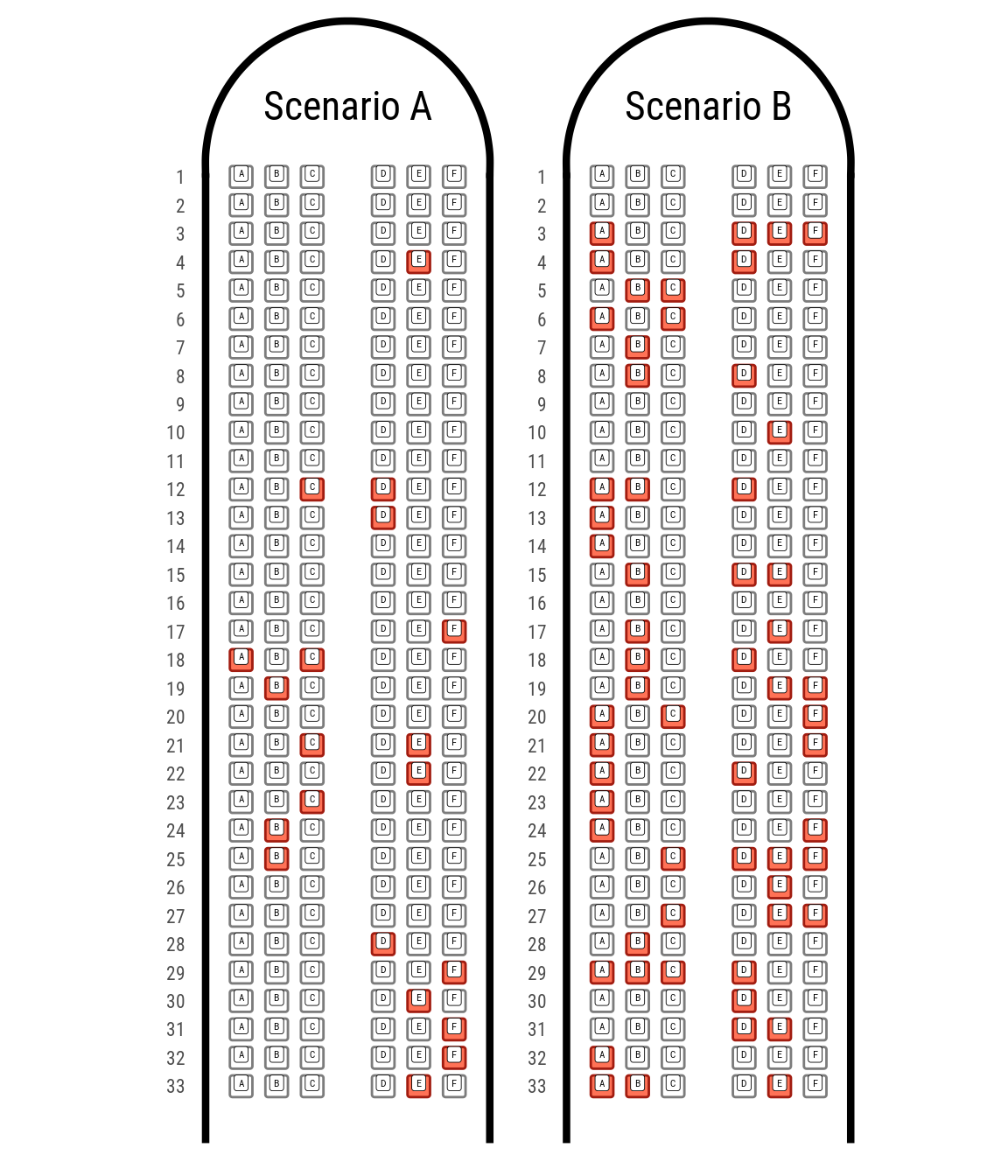If more than one airplane is needed:


rv_create_airplane_combo(risk_ratio = 0.01,
number_of_planes = 3,
font_family = "Roboto Condensed",
font_family_seats = "Roboto Mono", guides = NULL)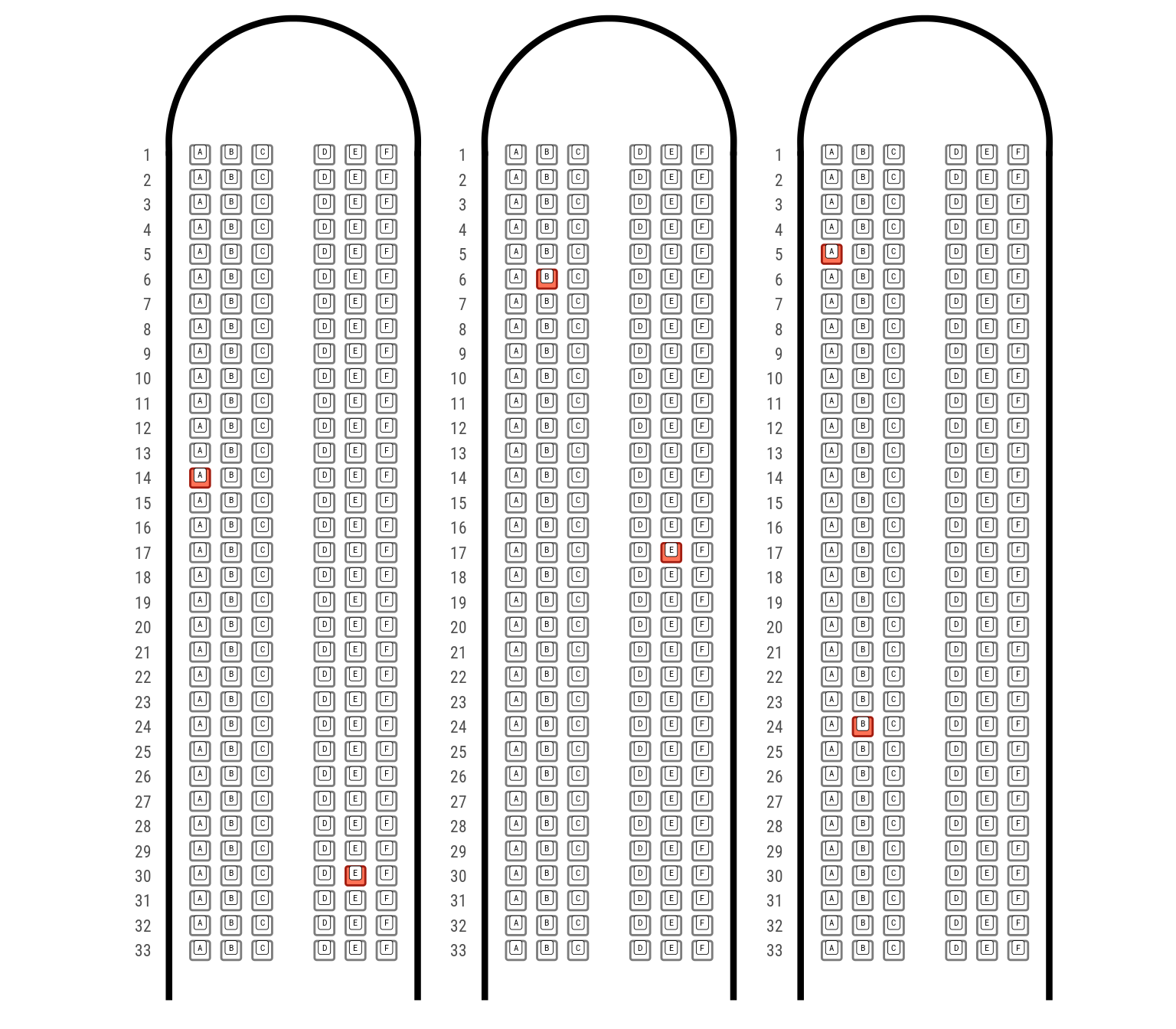rv_create_airplane_combo(risk_ratio = 0.001,
number_of_planes = 5,
font_family = "Roboto Condensed",
font_family_seats = "Roboto Mono",
legend_position = "none",
ncol = 3,
nrow = 2, guides = NULL)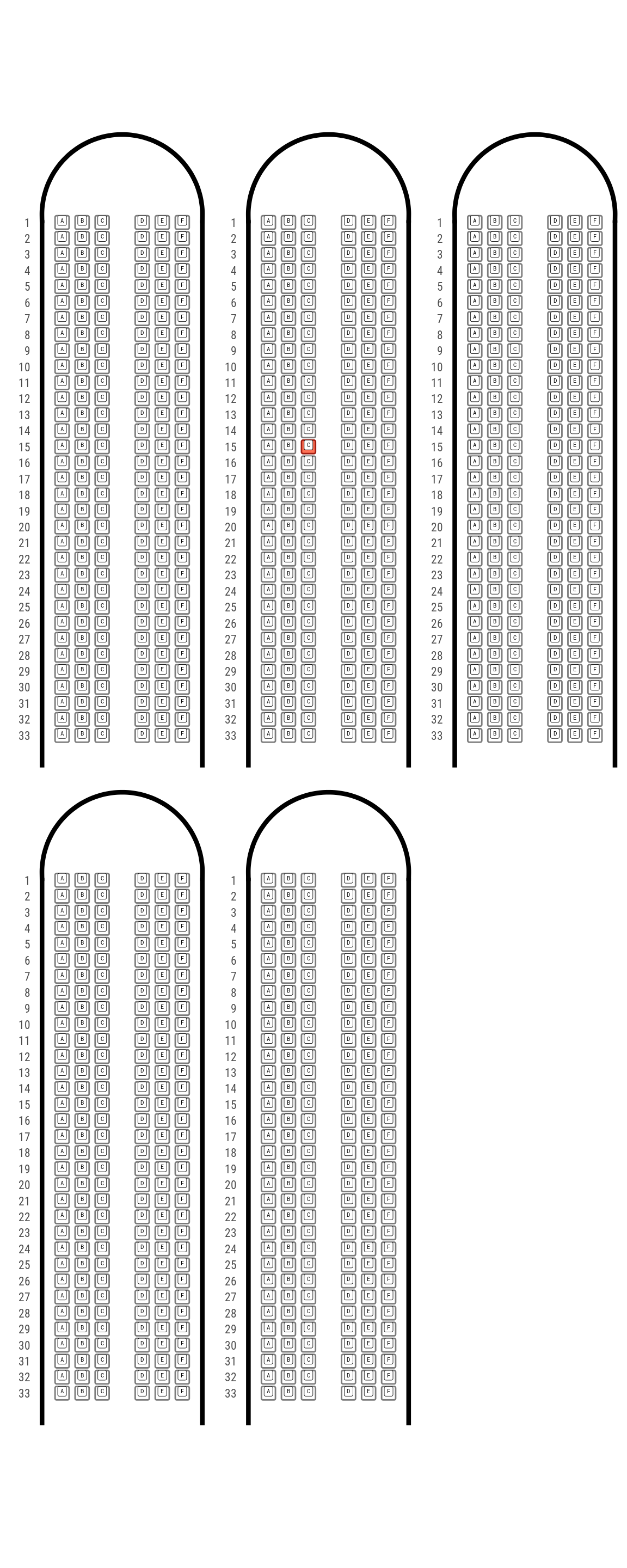Or, using more than one risk consideration:

risk_ratio <- tibble::tribble(~Risk, ~Ratio,
"Hospitalization", 0.3,
"Death", 0.1)

combo_gg <- rv_create_airplane_combo(risk_ratio = risk_ratio,
number_of_planes = 2,
font_family = "Roboto Condensed",
font_family_seats = "Roboto Mono",
legend_position = "bottom",
guides = "collect")

combo_gg +
patchwork::plot_annotation(title = "Risk caused by x for people aged xx",caption = 'Made with riskviewer, a tool by EDJNet',
theme = ggplot2::theme(plot.title = ggplot2::element_text(size = 24, family = "Roboto Condensed", hjust = 0.5)))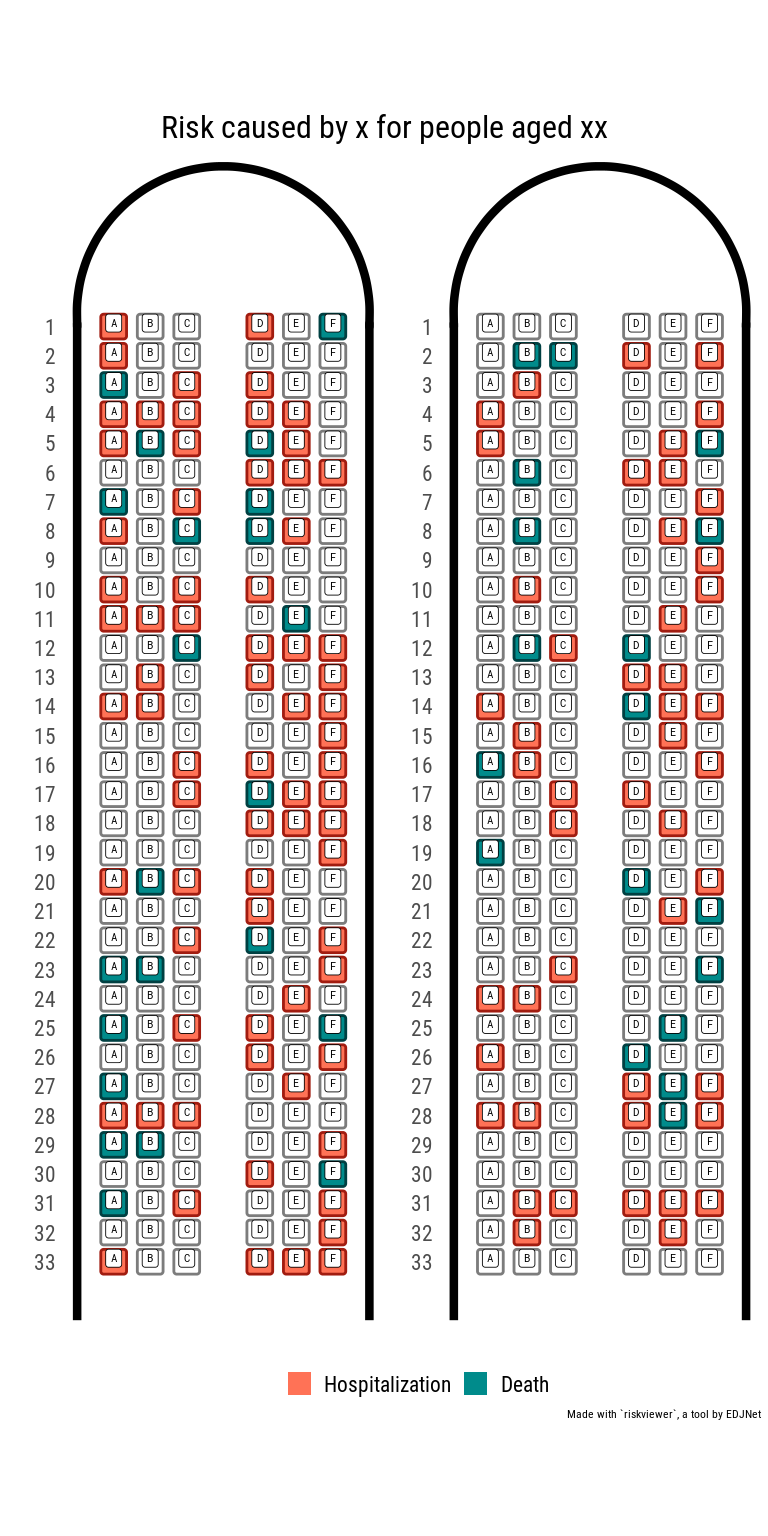## Compact airplane

combo_gg <- rv_create_airplane_combo(risk_ratio = risk_ratio,
number_of_planes = 2,
font_family = "Roboto Condensed",
font_family_seats = "Roboto Mono",
legend_position = "bottom",
guides = "collect",
compact = TRUE)

combo_gg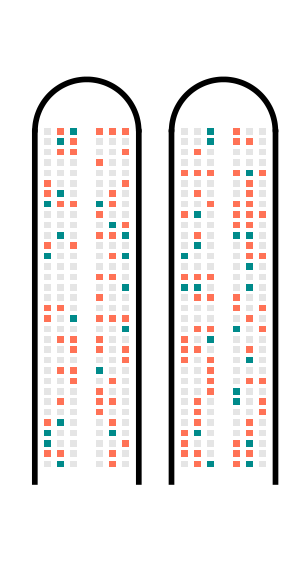The compact format makes it easier to have many planes:


rv_create_airplane_combo(risk_ratio = 0.002,
number_of_planes = 10,
compact = TRUE,
font_family = "Roboto Condensed",
legend_position = "none",
ncol = 5,
nrow = 2,
guides = NULL) +
patchwork::plot_annotation(title = "This risk hits about 2 people out of one thousand",caption = 'Made with riskviewer, a tool by EDJNet',
theme = ggplot2::theme(plot.title = ggplot2::element_text(size = 16, family = "Roboto Condensed", hjust = 0.5)))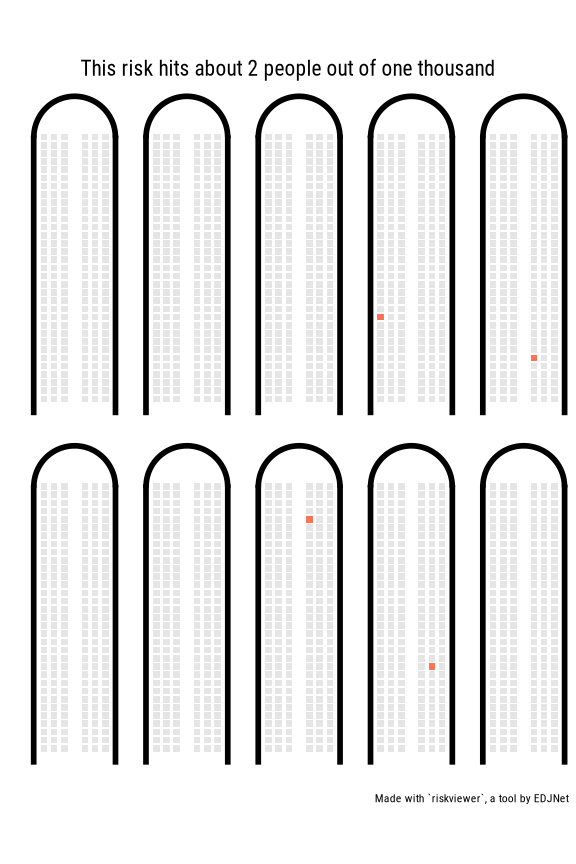## Animate transition between different risk scenarios

(currently broken)

gg_airplane_animated <- tibble::tribble(~Risk, ~Ratio,
"Age 20", 0.009,
"Age 30", 0.027,
"Age 40", 0.048,
"Age 50", 0.085,
"Age 60", 0.155,
"Age 70", 0.244,
"Age 80", 0.317) %>%
rv_create_airplane_animation(title = "Risk 1")

gganimate::animate(plot = gg_airplane_animated,
#renderer = gganimate::ffmpeg_renderer(),
height = 8,
width = 2,
units = "in",
res = 150)

### Arena

It is possible to create a ggplot object that looks like the seatmap of an arena, but this implies using two different projections, combining the graph, and leaving the top half empty.

library("riskviewer")
rv_create_arena(ratio = 0.05,
title = "The risk you face",
ggplot = FALSE,
quality = "low")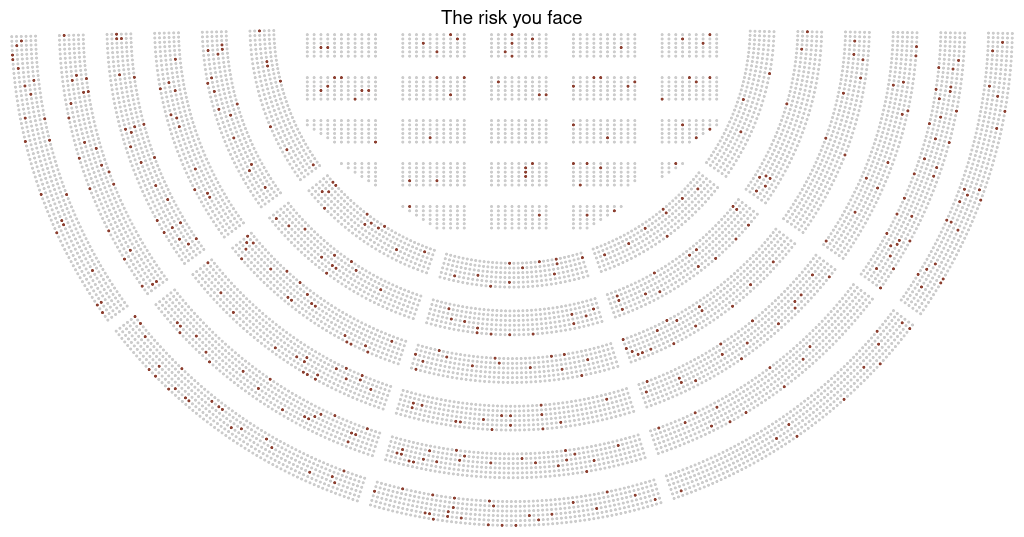library("riskviewer")
rv_create_arena(ratio = 0.3, ggplot = TRUE)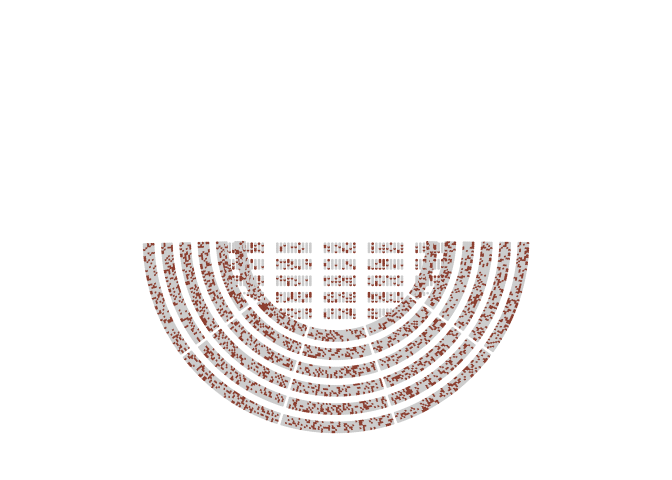## Combine more

graphs <- list(rv_create_arena(ratio = 0.1, title = "Scenario A", ggplot = TRUE),
rv_create_arena(ratio = 0.8, title = "Scenario B", ggplot = TRUE))

rv_img(plot = graphs, stack = TRUE)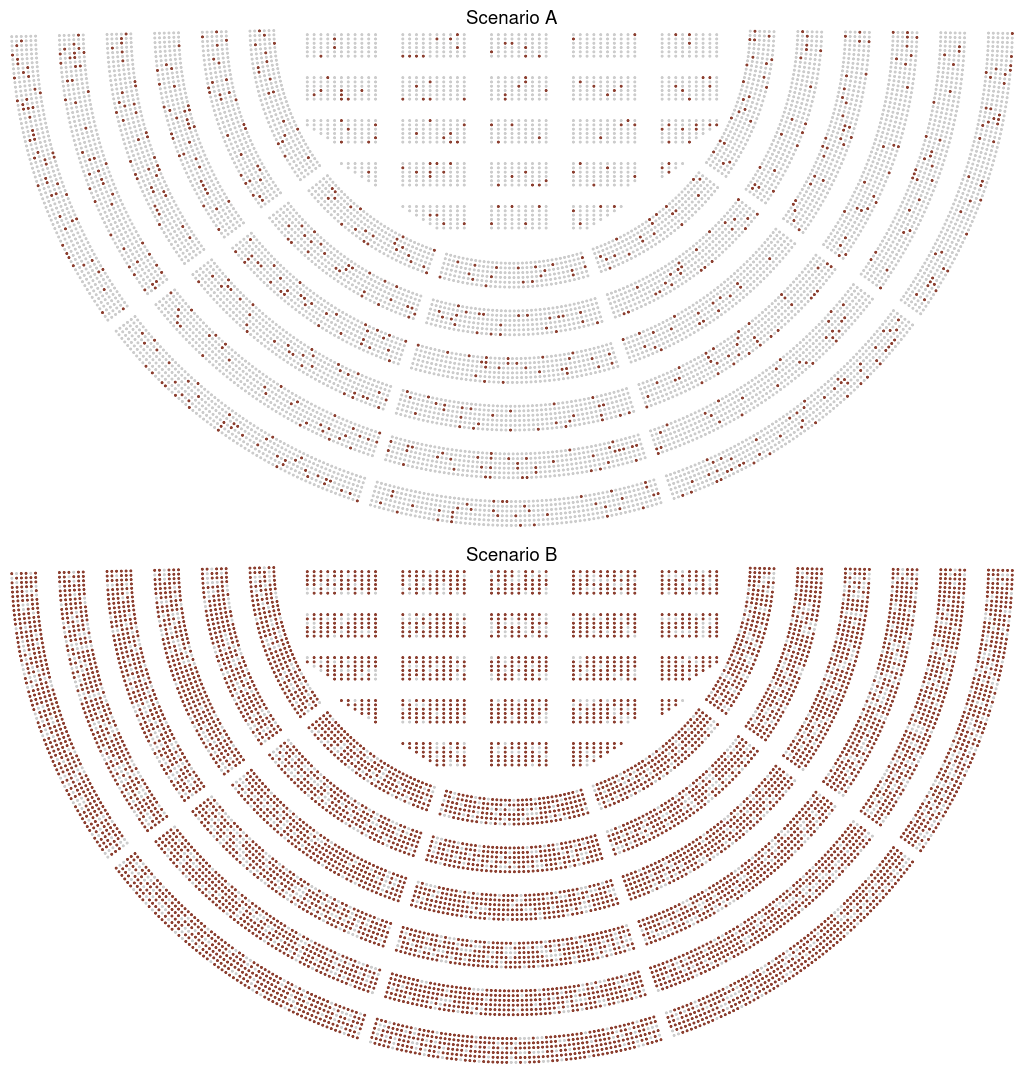rv_img(plot = graphs, stack = FALSE)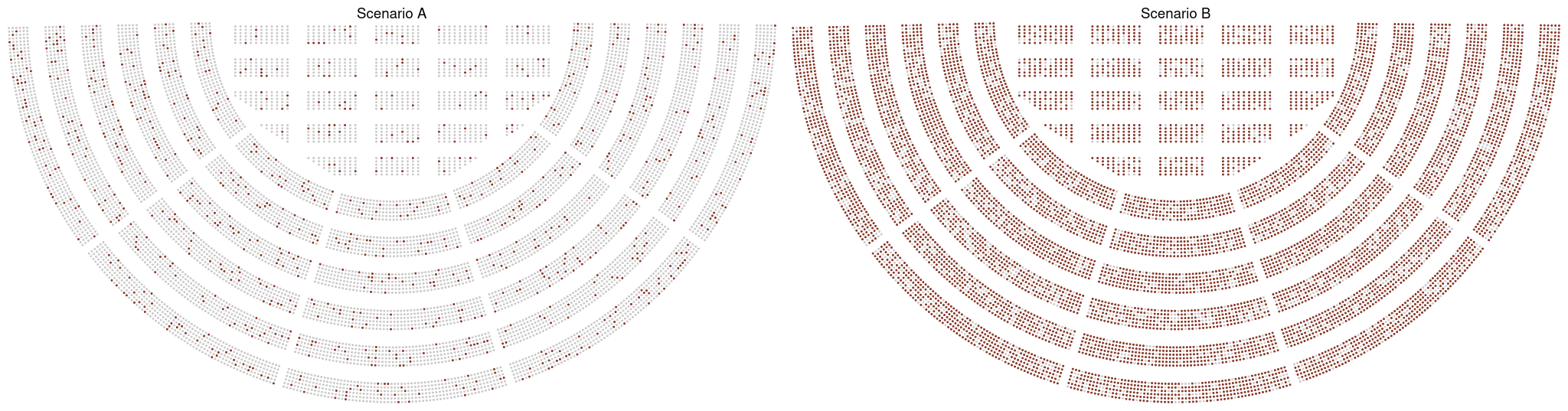## Export in other formats


ggplot2::ggsave(filename = "risk_arena.svg",
plot = rv_create_arena(ratio = 0.1, ggplot = TRUE),
width = 10,
height = 10,
units = "in")